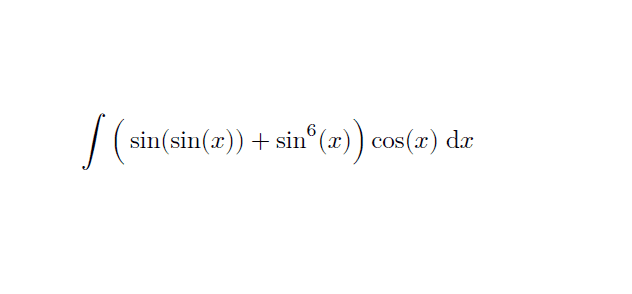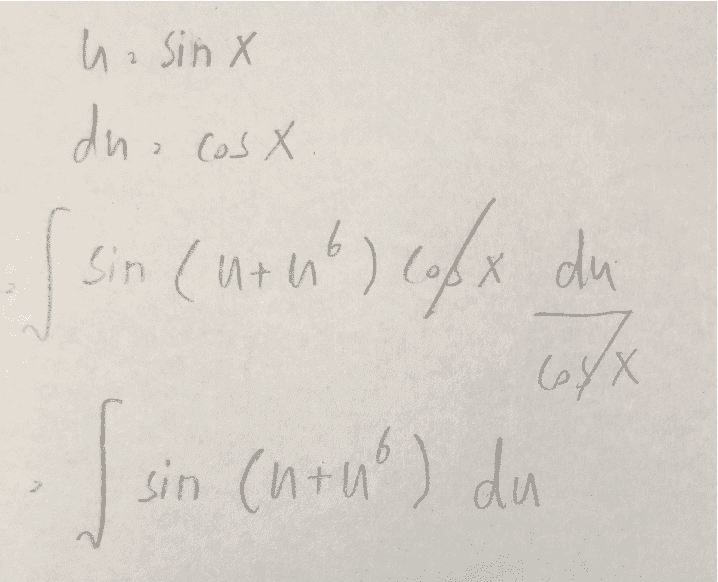# Hard integration problem

• MHB
jaychayI have tried to do it many times but still got the wrong answer.

Gold Member
MHB
Make the substitution $t = \sin x$.

jaychay
Make the substitution $t = \sin x$.I am stuck here
Get the parentheses in the right place! It's not $\sin(u+u^6)$, but $\sin(u) + u^6$, which is much easier to integrate.
Get the parentheses in the right place! It's not $\sin(u+u^6)$, but $\sin(u) + u^6$, which is much easier to integrate.